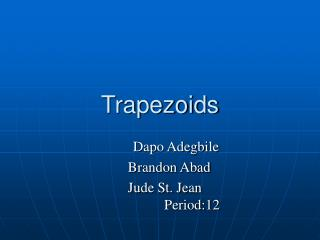DownloadDownload PresentationTrapezoids

# Trapezoids

Download Presentation## Trapezoids

- - - - - - - - - - - - - - - - - - - - - - - - - - - E N D - - - - - - - - - - - - - - - - - - - - - - - - - - -
##### Presentation Transcript

2. Definition • A quadrilateral that has 1 pair of parallel sides • A trapezoid with 1 pair of congruent sides

3. Properties of sides • The bases (top and bottom) of an isosceles trapezoid are parallel. • The legs of an isosceles trapezoid are congruent. • The angles on either side of the bases are congruent. • The bases (top and bottom) of a trapezoid are parallel. • Unlike the Isosceles Trapezoid it does not need to have any congruent sides.

4. Properties of angles • Adjacent angles along the sides are supplementary. • Base angles of isosceles trapezoid are congruent. • Normal trapezoids don’t have any special properties. • All of the angles within a trapezoid add up to 360 degrees.

5. Given Given Given Construction Construction Definition of a parallelogram Opposite sides of Parallelogram are congruent Transitive Property Isosceles Triangle Theorem (if sides than angles) Corresponding angles Transitive property Quad. ABCD is an Isosceles Trapezoid BC ll AD AB = DC Construct a line through C that is ll to AB AB ll EC Quad ABCE is a parallelogram AB = EC DC = CE <3 = <4 <1 = <3 <1 = <4 Proof Given: BC ll AD, AB = DC Prove: <1=<2

6. Properties of diagonals • The diagonals of an isosceles triangle are congruent. • Nothing special happens with the diagonals.

7. Quad. PQRS is a trapezoid SR ll PQ SP=RQ <SPQ=<RPQ PQ=PQ RP=SQ Given Given Given Definition of Trapezoid Reflexive Property CPCTC Proof Given: PQRS is an Isosceles Trapezoid SR ll PQ SP=RQ Prove: RP=SQ

8. Lines of symmetry • A regular trapezoid has no lines of symmetry • Isosceles trapezoids have only 1 line of symmetry

9. Coordinate Geometry • http://mathopenref.com/coordtrapezoid.html

10. formulas • Perimeter = a + b + c + B • Area = 1/2h(B+b) •    Area of parallelogram (B+b) x h   • But, this is double of what we need. So, multiply by 1/2. • The Area could also be altitude x median

11. Other facts • Right Trapezoid- a trapezoid with 2 right angles • median- is a line segment linking the midpoints of the two legs of the trapezoid • To find the length of the median you can find the length of the base and divide it by 2 or find the distance between the 2 midpoints of the legs • Altitude- is the perpendicular distance from one base to the other • British call it Trapezium

12. Suggested Websites http://mathopenref.com/coordtrapezoid.html http://www.coolmath.com/reference/trapezoids.html http://www.cliffsnotes.com/study_guide/Properties-of-Trapezoids.topicArticleId-18851,articleId-18798.html